Geometry

# Properties of Triangles: Level 2 Challenges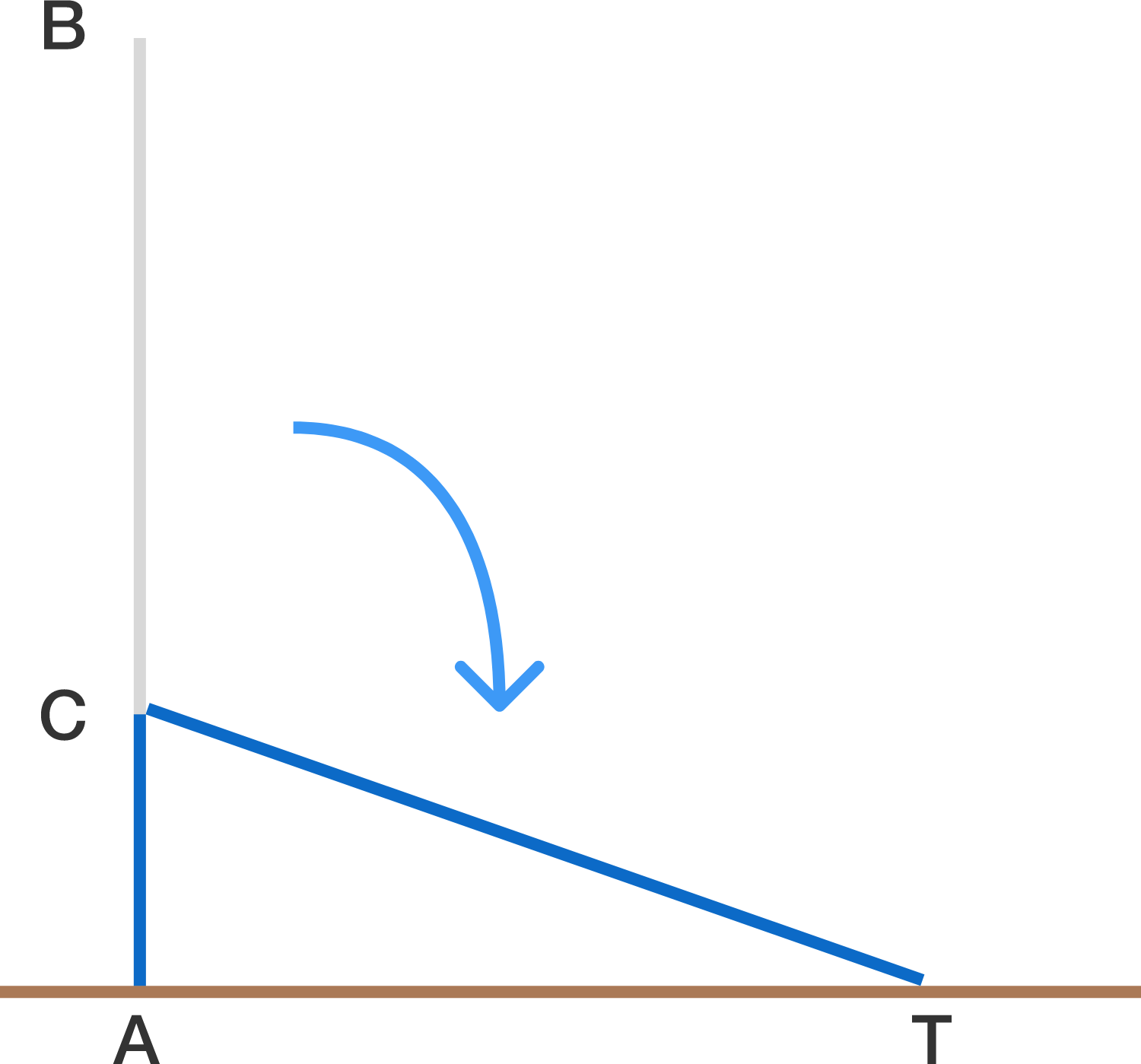A vertical pole AB measuring 5 meters snaps at point C. The pole remains in contact at C and the top of the pole touches the ground at point T, a distance of 3 meters from A.
Find the length AC, in meters, the point where the pole snapped.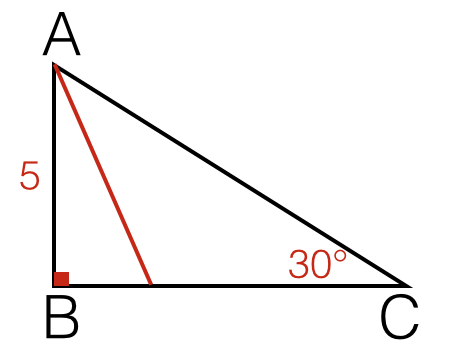Let $ABC$ be a triangle with $\angle B=90^\circ$, $\angle C=30^\circ$ and $AB=5$ Find the length of the angle bisector from $A$ to $\overline{BC}$(right to $2$ decimal places).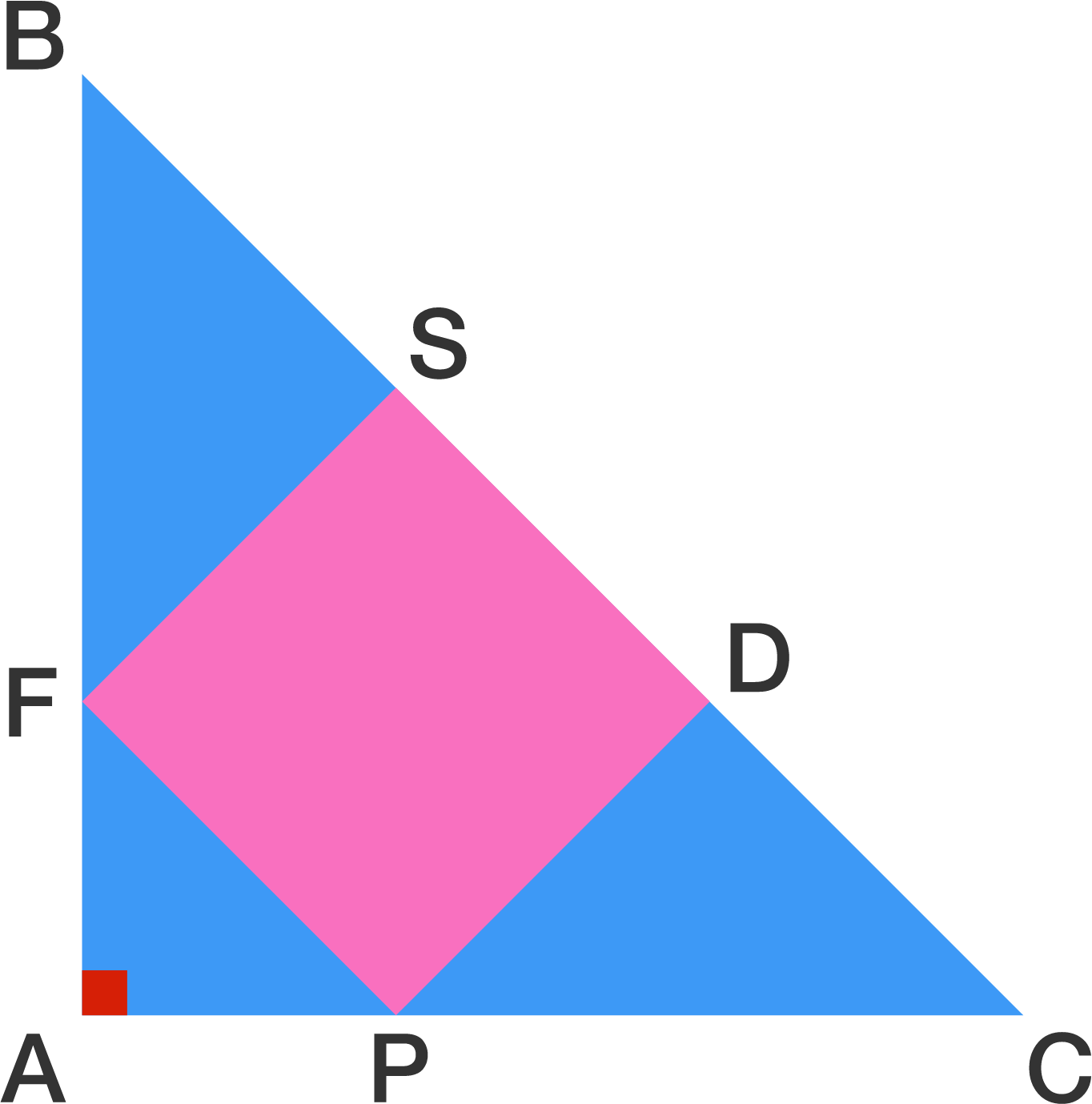$ABC$ is an isosceles right triangle with $AB = AC = 6$.

Given that $SDPF$ is a square, find the area of the square.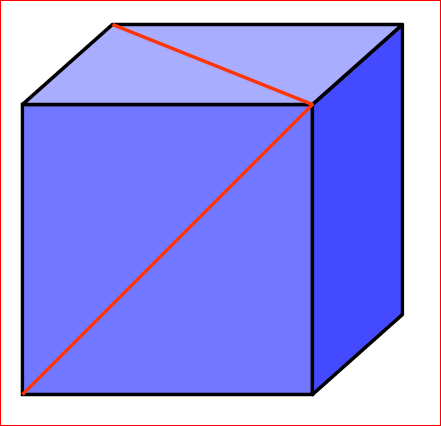The two red lines in the diagram shown are drawn diagonally on the faces of a cube. What is the angle (in degrees) between them at the point where they join?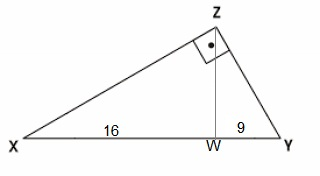In the image above, $\overline { ZW } \bot \overline { XY },$ $\overline { XW } =16$ and $\overline { WY } =9$. Find $\overline { ZY } +\overline {XY }$

×

Problem Loading...

Note Loading...

Set Loading...